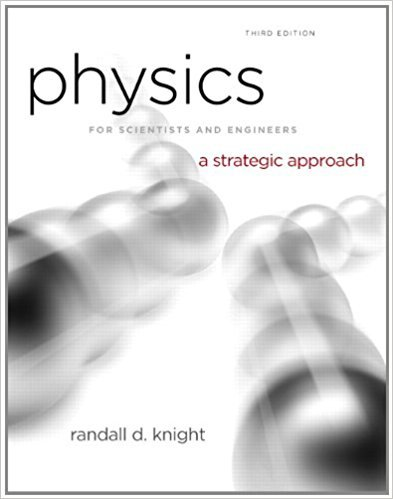×
Get Full Access to Physics For Scientists And Engineers: A Strategic Approach With Modern Physics - 3 Edition - Chapter 16 - Problem 59p
Get Full Access to Physics For Scientists And Engineers: A Strategic Approach With Modern Physics - 3 Edition - Chapter 16 - Problem 59p

×

# 0.10 mol of gas undergoes the process 1 ? 2 shown inISBN: 9780321740908 69

## Solution for problem 59P Chapter 16

Physics for Scientists and Engineers: A Strategic Approach with Modern Physics | 3rd Edition

• Textbook Solutions
• 2901 Step-by-step solutions solved by professors and subject experts
• Get 24/7 help from StudySoup virtual teaching assistantsPhysics for Scientists and Engineers: A Strategic Approach with Modern Physics | 3rd Edition

4 5 1 419 Reviews
26
0
Problem 59P

Problem 59P

0.10 mol of gas undergoes the process 1 → 2 shown in FIGURE.

a. What are temperatures T 1 and T 2 (in °C)?

b. What type of process is this?

c. The gas undergoes an isothermal compression from point 2 until the volume is restored to the value it had at point 1. What is the final pressure of the gas?

FIGUREStep-by-Step Solution:

Solution 59 P

a.)

Step 1 of 3

We have to find the temperaturesandof 0.1 mol of a gas which undergoes the processshown in the given figure.

The gas undergoes an isobaric process (constant pressure pressure) as the initial and final pressure (atm) are the same which can be seen from the figure.

The temperaturesandof the gas can be found using the ideal gas equation.Where,atm = 101300 Pacm3 = 1000m3(from figure)J/mol K

Thus,Where,atm = 101300 Pacm3 = 3000m3(from figure)J/mol K

Thus,Therefore, the temperaturesandare152 oC and 93o C respectively.

b.)

Step 2 of 3

Step 3 of 3

##### ISBN: 9780321740908

Unlock Textbook Solution